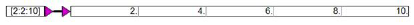## Filter operations

Filter operations can be represented mathematically by one or more difference equations. A general difference equation can be written as:This equation represents the relationship between the kth sample of the output to the N previous values of the output, the M previous values of the input, and the current value of the input. If all the coefficients bj are 0, the resulting filter is called a non-recursive or Finite Impulse Response (FIR) filter. Recursive filters are also known as Infinite Impulse Response (IIR) filters.

In FIR filters, the output is simply the weighted sum of the current and previous inputs. In contrast, in IIR filters, the output is the weighted sum of the current and previous inputs, and the previous outputs.